2022: SklogWiki celebrates 15 years on-line

# Gibbs-Duhem integration

The so-called Gibbs-Duhem integration refers to a number of methods that couple molecular simulation techniques with thermodynamic equations in order to draw phase coexistence lines. The original method was proposed by David Kofke  .

## Basic Features

Consider two thermodynamic phases: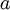$a$ and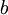$b$, at thermodynamic equilibrium at certain conditions. Thermodynamic equilibrium implies:

• Equal temperature in both phases: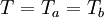$T = T_{a} = T_{b}$, i.e. thermal equilibrium.
• Equal pressure in both phases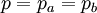$p = p_{a} = p_{b}$, i.e. mechanical equilibrium.
• Equal chemical potentials for the components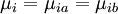$\mu_i = \mu_{ia} = \mu_{ib}$, i.e. material equilibrium.

In addition, if one is dealing with a statistical mechanical model, having certain parameters that can be represented as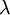$\lambda$, then the model should be the same in both phases.

## Example: phase equilibria of one-component system

Notice: The derivation that follows is just a particular route to perform the integration

• Consider that at given conditions of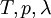$T , p, \lambda$ two phases of the systems are at equilibrium, this implies: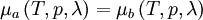$\mu_{a} \left( T, p, \lambda \right) = \mu_{b} \left( T, p, \lambda \right)$

Given the thermal equilibrium we can also write: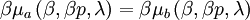$\beta \mu_{a} \left( \beta, \beta p, \lambda \right) = \beta \mu_{b} \left( \beta, \beta p, \lambda \right)$

where

•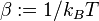$\beta := 1/k_B T$, where$k_B$ is the Boltzmann constant

When a differential change of the conditions is performed one will have, for any phase: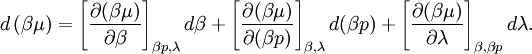$d \left( \beta\mu \right) = \left[ \frac{ \partial (\beta \mu) }{\partial \beta} \right]_{\beta p,\lambda} d \beta + \left[ \frac{ \partial (\beta \mu) }{\partial (\beta p)} \right]_{\beta,\lambda} d (\beta p) + \left[ \frac{ \partial (\beta \mu) }{\partial \lambda} \right]_{\beta,\beta p} d \lambda.$

Taking into account that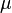$\mu$ is the Gibbs energy function per particle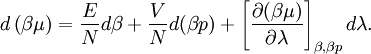$d \left( \beta\mu \right) = \frac{E}{N} d \beta + \frac{ V }{N } d (\beta p) + \left[ \frac{ \partial (\beta \mu) }{\partial \lambda} \right]_{\beta,\beta p} d \lambda.$

where:

•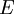$\left. E \right.$ is the internal energy (sometimes written as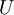$U$).
•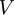$\left. V \right.$ is the volume
•$\left. N \right.$ is the number of particles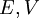$\left. \right. E, V$ are the mean values of the energy and volume for a system of$\left. N \right.$ particles in the isothermal-isobaric ensemble

Let us use a bar to design quantities divided by the number of particles: e.g.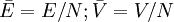$\bar{E} = E/N; \bar{V} = V/N$; and taking into account the definition: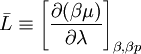$\bar{L} \equiv \left[ \frac {\partial (\beta \mu )}{\partial \lambda }\right]_{\beta,\beta p}$

Again, let us suppose that we have a phase coexistence at a point given by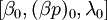$\left[ \beta_0, (\beta p)_0, \lambda_0 \right]$ and that we want to modify slightly the conditions. In order to keep the system at the coexistence conditions: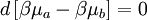$d \left[ \beta \mu_{a} - \beta \mu_b \right] = 0$

Therefore, to keep the system on the coexistence conditions, the changes in the variables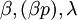$\beta, (\beta p), \lambda$ are constrained to fulfill: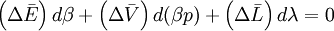$\left( \Delta \bar{E} \right) d \beta + \left( \Delta \bar{V} \right) d (\beta p) + \left(\Delta \bar{L} \right) d \lambda = 0$

where for any property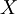$X$ we can define: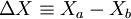$\Delta X \equiv X_a - X_b$ (i.e. the difference between the values of the property in the phases). Taking a path with, for instance constant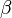$\beta$, the coexistence line will follow the trajectory produced by the solution of the differential equation: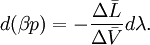$d(\beta p) = - \frac{ \Delta \bar{L} }{\Delta \bar{V} } d \lambda.$ (Eq. 1)

The Gibbs-Duhem integration technique, for this example, will be a numerical procedure covering the following tasks:

• Computer simulation (for instance using Metropolis Monte Carlo in the NpT ensemble) runs to estimate the values of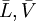$\bar{L}, \bar{V}$ for both

phases at given values of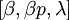$[\beta, \beta p, \lambda ]$.

• A procedure to solve numerically the differential equation (Eq.1)

## Peculiarities of the method (Warnings)

• The integrand of the differential equation is computed with some numerical uncertainty
• Care must be taken to reduce (and estimate) possible departures from the correct coexistence lines